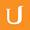## Implementing Dh Solution - Applied Cryptography

• 0:00 - 0:03
So the answer is we don't need 20 multiplications; we only need 4.
• 0:03 - 0:09
And the property we're taking advantage of is that X to the 2A is equal to X to the A squared.
• 0:09 - 0:13
That means we can break 2 to the 20 down into parts--
• 0:13 - 0:15
it's equal to 2 to the 10th squared
• 0:15 - 0:18
which is equal to 2 to the 5 squared squared
• 0:18 - 0:23
which is equal to--now 2 to the 5 is not divisible by 2--
• 0:23 - 0:30
so that's equal to 2 to the 4 times 2 squared and squared.
• 0:30 - 0:32
And so the number of multiplications--
• 0:32 - 0:35
well what we're computing is each square involves a multiplication--
• 0:35 - 0:39
so there's 1 multiplication there, there's 1 multiplication there,
• 0:39 - 0:43
there's 1 multiplication there, and there's 1 multiplication there--
• 0:43 - 0:44
so 4 total multiplications.
• 0:44 - 0:48
So this does not scale exponentially in the size of the power.
• 0:48 - 0:51
So this means computing A to the N power requires
• 0:51 - 0:54
on the order of log N multiplications.
• 0:54 - 0:56
In terms of the size of N, that means it's linear
• 0:56 - 1:00
in terms of the number of bits needed to represent the power.
Title:
Implementing Dh Solution - Applied Cryptography
Video Language:
English
Team:Udacity
Project:
CS387 - Applied Cryptography
Duration:
01:33Udacity Robot edited English subtitles for Implementing Dh Solution - Applied CryptographyGundega added a translation

# English subtitles

## Revisions Compare revisions

• API
Udacity Robot
• Gundega